Deutsche Version● What is Amplitude?
Definition of Amplitude in the Terms of Sound

Good question: What is meant by amplitude?
What is the term commonly used to
describe a sound wave's amplitude?
How to calculate amplitude?
What is amplitude measured in?
There are four terms describing a
sound wave:
wavelength, period, frequency, and amplitude

 Amplitude Definition: 1.The maximum extent of a vibration or displacement of a sinusoidal (!) oscillation, measured from the position of equilibrium. Amplitude is the maximum absolute value of a periodically varying quantity. 2.The maximum difference of an alternating electrical current or potential from the average value.   The term "amplitude" is used to refer to the magnitude of an oscillation, so the amplitude of the sinusoid "y = A × sin (ω×t)", is | A |, where | A | is the absolute value of A.   The amplitude is a variable characterizing a sinusoidal oscillation. It gives the deflection of a physical quantity from its neutral position (zero point) up to a positive or negative value. The amplitude is expressed in a physical quantity − for example, as voltage, sound pressure, etc. Amplitudes are expressed either as instantaneous values or mostly as peak values. Amplitude is the fluctuation or displacement of a wave from its mean value. With sound waves, it is the extent to which air particles are displaced, and this amplitude of sound or sound amplitude is experienced as the loudness of sound. From the "Encyclopedia Britannica": For a transverse wave, such as the wave on a plucked string, amplitude is measured by the maximum displacement of any point on the string from its position when the string is at rest. For a longitudinal wave, such as a sound wave, amplitude is measured by the maximum displacement of a particle from its position of equilibrium. When the amplitude of a wave steadily decreases because its energy is "being lost" (converted to heat), it is said to be damped. Sound waves in air are longitudinal, pressure waves.Drop a stone on a pond

 Commonly it is spoken of "amplitude", as if there would be just a certain amplitude as displacement or elongation from the zero-axis (baseline or equilibrium). Amplitude can be a word that describes a wave. It means that maximum amount the wave varies from the baseline or equilibrium. Displacement is usually used to describe particles in motion, as in how far a particle has moved from a given point. The wavelength in a longitudinal wave refers to the distance between two consecutive compressions or between two consecutive rarefactions. Definition: The amplitude is the maximum displacement from equilibrium. For a longitudinal wave which is a pressure wave this would be the maximum increase (or decrease) in pressure from the equilibrium pressure that is cause when a compression (or rarefaction) passes a point. The amplitude is the distance from the equilibrium position of the medium to a compression or a rarefaction. The peak value of sinusoidal AC signals is referred to as amplitude starting from the zero line. The amplitude usually refers to the scalar or vector field size.

Wavelength and DistancePeriod and Time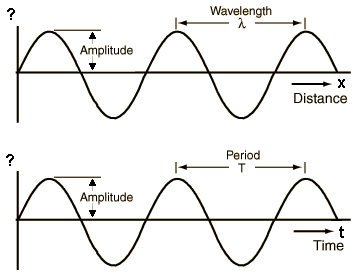The amplitude A has nothing to do with frequency, wavelength, period of time, and speed of sound.

 There is not only one amplitude. There are many amplitudes. Look at: "Soundfield Quantities of a Plane Wave" http://www.sengpielaudio.com/SoundfieldQuantitiesPlaneWaveAmplitude.pdf

 We often hear the question: How big is the amplitude? In our case, it is the "sound amplitude". Usually, we are asking as if there is just "one" amplitude of sound waves in air. The loudness perception of a sound is determined by the amplitude of the sound waves − the higher the amplitude, the louder the sound or the noise. Which amplitude of sound (sound amplitude)? In the above link "Soundfield Quantities of a Plane Wave" we find the: amplitude of particle displacement ξ, or displacement amplitude amplitude of sound pressure p or pressure amplitude amplitude of sound particle velocity v, or particle velocity amplitude amplitude of pressure gradient Δ p, or pressure gradient amplitude.   Every time we add 6 dB, actually the amplitude of the signal is doubled.   All these terms are sound field quantities. There are problems with sound energy quantities (power), when we use the term amplitude. Avoid any applying of the word amplitude at power levels or energy quantities. Sound field quantity is not a sound energy or sound power quantity.   Furthermore, think of the amplitude of the oscillation of a string. The maximum magnitude of the deflection of a wave is called amplitude.   Harmonic motion: Displacement = A × sin (2 × π × f × t), that means: A = amplitude (peak), f = frequency, t = time.   Sound particle velocity v should not be confused with velocity of sound c or speed of sound, all are measured in m/s:

 Amplitude as particle displacement ξ = v / (2 π × f ) = p / (2 π × Z) Pressure amplitude = Amplitude as sound pressure p = ξ × 2 π × Z = v × Z        Z = ρ × c Specific acoustic impedance of air at 20°C is Z = 413 N·s/m³   The sound pressure amplitude is the maximum value of the sound pressure. Since the sound pressure p is a periodic quantity, it is specified as effective sound pressure pRMS (root mean square).

 The change in the amplitude has nothing to do with the change in pitch (frequency) and vice versa.

 Amplitudes, correlated   Voltage sum, coherent (0°)   1 + 1 = 2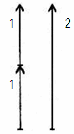Amplitudes, uncorrelated Power sum, incoherent (90°) √ (1² + 1²) = 1.414 ...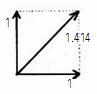The sound intensity is proportional to the amplitude (sound pressure) squared; I ~ p², so amplitude (sound pressure) is proportional to the square root of sound intensity; p ~ √ I.

What is an amplitude?
http://www.indiana.edu/~emusic/acoustics/amplitude.htm

 http://uk.answers.yahoo.com/question/index?qid=20111025210805AAIaFhX Question of answers.yahoo:   Sound... What is amplitude? I'm wondering what is it that creates the amplitude of a sound wave?   I understand that as represented as a transverse wave, amplitude is the maximum value of the wave function but how does this translate into longitudinal waves? It makes sense to me that the shorter or further the distance between a high or low pressure pocket of air makes the difference between a higher or lower frequency sound but seeing as the frequency determines the pitch and the speed of sound is constant (depending on the medium) what is it that provides a softer or louder sound? I've read that the intensity or energy of the sound waves is what makes it louder or quieter, but if sound is travelling at the same speed, what property of the wave as it travels through air is the term intensity or energy referring to?

 Answer of answers.yahoo:   "Sound... What is amplitude?" That is a really good question, because there is a problem with the definition of the word amplidude. Amplitude is the magnitude of change in the oscillating variable with each oscillation within an oscillating system. For example, sound waves in air are oscillations in atmospheric pressure and their amplitudes are proportional to the change in pressure during one oscillation. If a variable undergoes regular oscillations, and a graph of the system is drawn with the oscillating variable as the vertical axis and time as the horizontal axis, the amplitude is visually represented by the vertical distance between the extrema of the curve and the equilibrium value. In older texts the phase is sometimes very confusingly called the amplitude.   Particle displacement is called particle amplitude. A transverse wave has an amplitude. Particle velocity has an amplitude. Sound pressure or acoustic pressure has an amplitude. Every (audio) frequency has an amplitude. A pendulum has an amplitude.   Disputable information: "Sound intensity or acoustic intensity has an amplitude. Sound power has an amplitude. Sound energy has an amplitude. Sound energy density has an amplitude. Sound energy flux has an amplitude." But these are sound energy sizes. The amplitude does not show directly the energy - The greater the amplitude the greater is the energy. Energy = amplitude squared.   So what is amplitude? A "sound" has an amplitude. A loud sound has a bigger amplitude than a soft sound. Which amplitude is really meant?   Cheers ebs

 http://answers.yahoo.com/question/index?qid=20111003100847AAP1uuF Question of answers.yahoo:   Loudness of sound depends upon amplitude or frequency? Also tell the relation between them.

 Answer of answers.yahoo:   Loudness depends on sound pressure, frequency, bandwidth, and duration. Loudness is the quality of a sound that is primarily a psychological correlate of physical strength (amplitude). It is a subjective measure, often confused with objective measures of sound strength such as sound field sizes, like sound pressure or sound pressure level SPL in decibels, and sound energy sizes like sound intensity or sound power; see: "Loudness - Wikipedia": http://en.wikipedia.org/wiki/Loudness   Cheers ebs   PS: Don't forget that our eardrums are effectively moved by the "sound pressure"; see: "Sound pressure and Sound power − Effect and Cause": http://www.sengpielaudio.com/SoundPressureAndSoundPower.pdf   We measure the sound by an SPL meter (SPL = Sound Pressure Level).

 Note: Time, frequency and phase belong close together. The height of the amplitude has no influence on those parameters.   The amplitude A has nothing to do with the frequency, the wavelength, the time duration and the speed of sound.
 The waveform parameters of a "117 V and 230 V RMS alternating current" sine wave form are summarized at the table below.

 Average voltage RMS voltage (VRMS) Peak voltage (Vp) = (Û) Peak-to-peak voltage (Vpp) 0 volts 117 volts = VRMS = ~V 165 volts = √2×VRMS = 0,5 × Vpp 330 volts = 2×√2×VRMS = 2 × Vp 0 volts 230 volts = VRMS = ~V 325 volts = √2×VRMS = 0,5 × Vpp 650 volts = 2×√2×VRMS = 2 × Vp

 The value VRMS of an alternating voltage V (t) = V0 × f(t) is defined so that the effective DC power corresponds VRMS2 / R = VRMS × IRMS to an ohmic resistance of the middle resistive power of this AC voltage to the same resistance.

 Enter one known voltage: VRMS = Volt Vp      = Volt Vpp    = Volt Do not reenter an answer!The crest factor means the ratio of the peak voltage to the RMS voltage. If we need to calculate an attenuator (attenuation calculation) we calculate a voltage divider.

Voltage conversions

 VRMS = ~V Vp Vpp Average voltage RMS VRMS  = − 0.7071 × Vp 0.3535 × Vpp Peak voltage Vp   = 1.414 × VRMS − 0.5000 × Vpp Peak-to-peak voltage   Vpp = 2.828 × VRMS 2.000 × Vp −

Unclear equations in books

 The sound intensity I in W/m2 in a plane progressive wave is given as:or also as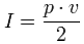But only one equation can be correct.   Sometimes, these equations will show further information: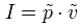or also as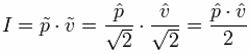The tilde will indicate that it is the RMS value and the roof will show that it is the amplitude value, ie, the peak value. For sinusoidal signals, the peak value means the amplitude. With these more accurate data, both equations are correct. You just need to know exactly whether the peak value or the RMS value is applied. Sound intensity = sound pressure × particle velocity Sound intensity = (force / area) × (particle displacement / time) Sound intensity = sound energy / (area × time) = sound power / area. I = p × v = (F / A) × (ξ / t) = E / (A × t) = Pac / A.   Sound pressure p in Pa = N/m2 – particle velocity v in m/s − acoustic intensity I in W/m2 that is N/m2 · m/s                Energy equivalent: J (joule) = N·m = W·s   In audio engineering we always (!) assume RMS values for sound field quantities (sizes), if not specially noted different. The reference sound pressure is p0 = 20 µPa = 2 × 10−5 Pa (threshold of hearing) and this is the RMS value.

 Sound waves are longitudinal waves in the air that we perceive as vibration: sound pressure amplitude, sound intensity, sound energy, tonality, impulsiveness, sharpness, loudness, volume, annoyance, roughness, brittleness, echo content, clarity, intelligibility, information content.